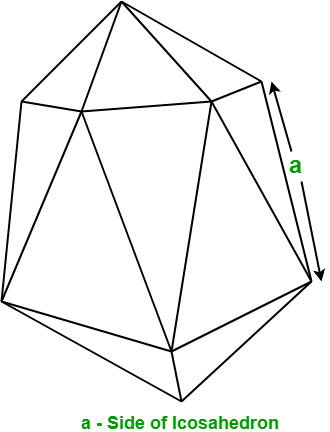Related Articles
Program to find the Area and Volume of Icosahedron
• Difficulty Level : Medium
• Last Updated : 17 Mar, 2021

Given the side of an Icosahedron. The task is to find the area and volume of the given Icosahedron.
Examples

Input : a = 5
Output : Area: 216.506
Volume: 272.712

Input : a = 10
Output : Area: 866.0254
Volume: 2181.695

In geometry, an Icosahedron is a regular polyhedron which contains 20 identical equilateral triangular faces, 30 sides, and 12 vertices.Formula to find Area and Volume of Icosahedron: Let a be the side of Icosahedron, then

Surface area of Icosahedronand, Volume of Icosahedron## C++

 // C++ program to find the Area and// volume of Icosahedron#include using namespace std; // Function to find area of Icosahedronfloat findArea(float a){    float area;     // Formula to calculating area    area = 5 * sqrt(3) * a * a;         return area;} // Function to find volume of Icosahedronfloat findVolume(float a){    float volume;     // Formula to calculating volume    volume = ((float)5 / 12) * (3 + sqrt(5)) * a * a * a;         return volume;} // Driver Codeint main(){    float a = 5;     // Function call to find area of Icosahedron.    cout << "Area: " << findArea(a) << endl;         // Function call to find volume of Icosahedron.    cout << "Volume: " << findVolume(a);     return 0;}

## Java

 // Java program to find the Area and// volume of Icosahedronimport java.io.*; class GFG {         // Function to find area of Icosahedron    static float findArea(float a)    {        float area;             // Formula to calculating area        area = (float)(5 * Math.sqrt(3) * a * a);                 return area;    }         // Function to find volume of Icosahedron    static float findVolume(float a)    {        float volume;             // Formula to calculating volume        volume = (float)(((float)5 / 12) * (3 + Math.sqrt(5)) * a * a * a);                 return volume;    }         // Driver code    public static void main (String[] args)    {        float a = 5;         // Function call to find area of Icosahedron.        System.out.println("Area: " + findArea(a));                 // Function call to find volume of Icosahedron.        System.out.println("Volume: " + findVolume(a));    }}

## Python3

 # Python3 program to# find the Area and# volume of Icosahedron # import math module# to use sqrt functionfrom math import sqrt # Function to find# area of Icosahedrondef findArea(a):     # Formula to calculate area    area = 5 * sqrt(3) * a * a    return area # Function to find# volume of Icosahedrondef findVolume(a):         # Formula to calculate volume    volume = ((5 / 12) *              (3 + sqrt(5)) *               a * a * a)    return volume # Driver Codea = 5 # Function call to# find area of Icosahedron.print("Area: " , findArea(a))     # Function call to find# volume of Icosahedron.print("Volume: " , findVolume(a)) # This code is contributed# by ihritik

## C#

 // C# program to find the Area and// volume of Icosahedronusing System; public class GFG {         // Function to find area of Icosahedron    static float findArea(float a)    {        float area;             // Formula to calculating area        area = (float)(5 * Math.Sqrt(3) * a * a);                 return area;    }         // Function to find volume of Icosahedron    static float findVolume(float a)    {        float volume;             // Formula to calculating volume        volume = (float)(((float)5 / 12) * (3 + Math.Sqrt(5)) * a * a * a);                 return volume;    }         // Driver code    static public void Main ()    {        float a = 5;         // Function call to find area of Icosahedron.        Console.WriteLine("Area: " + findArea(a));                 // Function call to find volume of Icosahedron.        Console.WriteLine("Volume: " + findVolume(a));        //Code    }}

## PHP

 

## Javascript

 
Output:
Area: 216.506
Volume: 272.712

Attention reader! Don’t stop learning now. Get hold of all the important mathematical concepts for competitive programming with the Essential Maths for CP Course at a student-friendly price. To complete your preparation from learning a language to DS Algo and many more,  please refer Complete Interview Preparation Course.

My Personal Notes arrow_drop_up